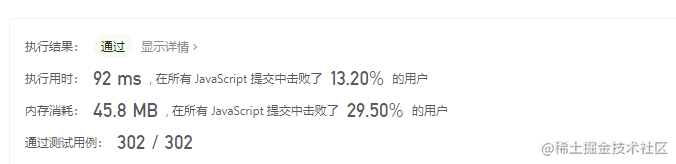# 1417. 重新格式化字符串

### 一、题目描述：

``````示例 1：

### 二、思路分析：

#### 思路

1、切割成数组（split），分成数字和字母分成两个数组

2、判断是否符合格式化的条件

3、遍历拼接

### 三、AC 代码：

``````/**

* @param {string} s

* @return {string}

*/

var reformat = function(s) {
// 切割成数组
const list = s.split('');
const charList = [];
const numList = [];
for(let i = 0; i < s.length; i += 1) {
// 判断是否不是number
if (isNaN(s[i])) {
charList.push(s[i]);
} else {
numList.push(s[i]);
}
}
// 判断数字和字母的长度绝对值是否大于1，如果是，则返回空字符串
if (Math.abs(charList.length - numList.length) > 1) {
return '';
}
// 拼接
let result = '';
let minLen = Math.min(numList.length, charList.length);
for (let i = 0; i < minLen; i++) {
result += numList[i] + charList[i];
}
numList.length > charList.length ? result += numList[numList.length - 1] : null;
numList.length < charList.length ? result = charList[charList.length - 1] + result : null;
return result;

};

### 四、总结：